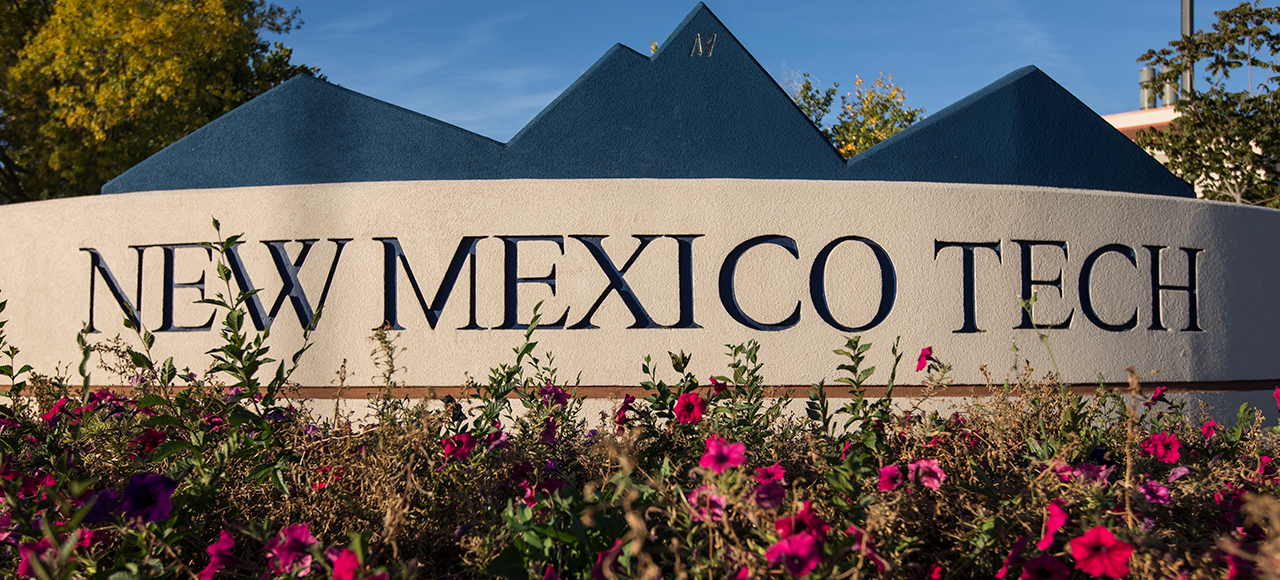Mathematics

# Faculty Research Areas

### Rakhim Aitbayev

Many phenomena in sciences and engineering are described by partial differential equations (PDEs). Exact solutions of most of real world problems are often very difficult or impossible to obtain. Solutions to such problems can be approximated using numerical methods. Numerical Methods for Solving PDEs is a vast area which deals with numerical errors, computational stability, parallel algorithms, efficient computation, and solution of challenging multi-physics problems. My research interests are in the following areas of Numerical PDEs: orthogonal spline collocation method, iterative methods for solving large sparse systems of equations, numerical solution of nonlinear elliptic PDEs, numerical solution of non-self-adjoint or indefinite problems, and interface problems.

### Ivan Avramidi

I work on problems at the intersection of global analysis on manifolds, Riemannian geometry and mathematical physics. The main part of my research is devoted to the study of spectral asymptotics of elliptic partial differential operators on manifolds via heat kernel methods and to the application of these methods to various problems of spectral geometry, mathematical physics, quantum field theory, quantum gravity and financial mathematics.

### Brian Borchers

My research interests are in optimization and applications of optimization techniques in inverse problems.  In optimization my research has involved interior point methods for linear and semi-definite programming with applications in integer programming.  The work on parameter estimation and inverse problems has involved optimization, statistics, and Tikhonov regularization.

### Gilberto Gonzalez-Parra

My main research interests are in the area of mathematical modeling. In particular, I have been involved in the mathematical modeling of processes that emerge from life sciences such as epidemics, diseases, social behaviors, viruses and cellular systems. It is important to remark that, life sciences are expanding and the related topics are increasing exponentially.  The life sciences problems nowadays have become a huge source of mathematical problems for different areas of mathematics. Thus, it has been necessary to create interdisciplinary groups to solve the challenge problems that arise. The main mathematical tools that I have been using for research are nonlinear dynamical systems, differential equations, numerical methods, discrete mathematics, probability, stochastic processes, scientific computing, and numerical analysis. In particular I have applied these tools to study the dynamics of influenza, Chagas, respiratory syncytial virus (RSV), Chikungunya, Toxoplasmosis, obesity, crime, rumors and others.

### Anwar Hossain

My research focuses mostly on diagnostic methods and their applications to reliability and engineering models. These two areas are crucial within the statistical research community, and are also favorable at technical institutions. In the past few years, I have focused on other fields of statistics; the most attention has been given to cover theory and applications of multivariate analysis, survival estimation, Bayesian estimation, and reliability.

### Bert Kerr

My research focuses on determining analytic solutions of Boundary Value Problems. For example, the types of mixed BVP's which arise in analyzing the stress field in the vicinity of a crack, or in the study of flow past an obstruction. I am currently working with the Electrical and Microsystems modeling group at Sandia National Lab. I work on solving BVP's, referred to as compact models. The development of compact models that can simulate the excess carrier dynamics in undepleted semiconductor regions are important for many applications, including photonic and power devices.

### Oleg Makhnin

Modeling complex multidimensional data; in particular, spatial data. Precipitation modeling. Geostatistics. Bioinformatics. Statistical consulting.

### W.D. Stone

My research interests are Mathematical Biology and Mathematical Modeling. Recently I have been increasingly working with modeling of Fluids. In the past few years I have had students working on modeling of glaciers, size-structured populations, age-distribution of groundwater, resonances in the plasmosphere, and other areas of modeling.